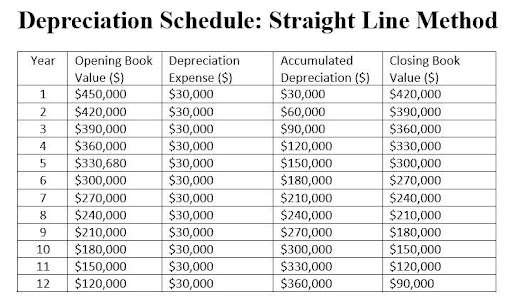## Instead of calculating each ratio individually

Let our ratio tool instantly calculate your ratios. Input your financial data once and get multiple results.

## Straight line depreciation

Straight line depreciation =

(Cost of asset – Salvage Value)

Useful Life

### INTERPRETATION

The straight line method calculates the depreciation expense for each asset and allocates the cost of each asset over the useful life.

Business owners, managers, and other interested parties use it to record the depreciation of assets whose book value steadily drops over time.

Note: expanded calculation

Spread the expenses of the asset evenly over its useful life after subtracting the asset’s salvage value.

### EXAMPLE

M&M purchased a grinder machine for \$450,000. It has an estimated salvage value of \$90,000 and a useful life of 12 years. M&M calculates the annual straight-line depreciation.

Description of asset: Grinder Machine

Year (start): 20XX

Cost of asset: \$450,000

Residual value (salvage value): \$90,000

Useful Life: 12 years

Depreciation Rate: 8.33%

Depreciable Cost: \$360,000

### SEE: Depreciation Schedule - Straight Line Method

Depreciation Rate = 100% / useful life

Depreciation Rate = 100% /12 years = 8.33%

Depreciable Cost = cost of asset – residual value

Depreciable Cost = \$450,000 – \$90,000 = 360,000

Depreciation Expense = Depreciation Rate x Depreciable Cost

Depreciation Expense = 8.33% x 360,000 = \$30,000

Accumulated Depreciation = Depreciation Expense + Accumulated Depreciation

Accumulated Depreciation (year 2) =\$30,000 + \$30,000 = \$60,000 in year 2

Closing Book Value = Opening Book Value – Depreciation Expense

Closing Book Value = \$450,000 – \$30,000 = \$420,000

### BENCHMARK: ROT

This method is used to calculate depreciation on machinery that you expect to use until you scrap it.Straight line depreciation :

### ABBREVIATION KEY:

ROT: Rule of thumb
HA: Historical Average (organization’s historical average)
PG: Peer Group average
EB: Economic Benchmark

DISCLAIMER: The interactive calculators on this site are self-help tools intended to help you visualize and explore your financial information. They are not intended to replace the advice of a qualified professional. Because each business is different, we can not guarantee accuracy.